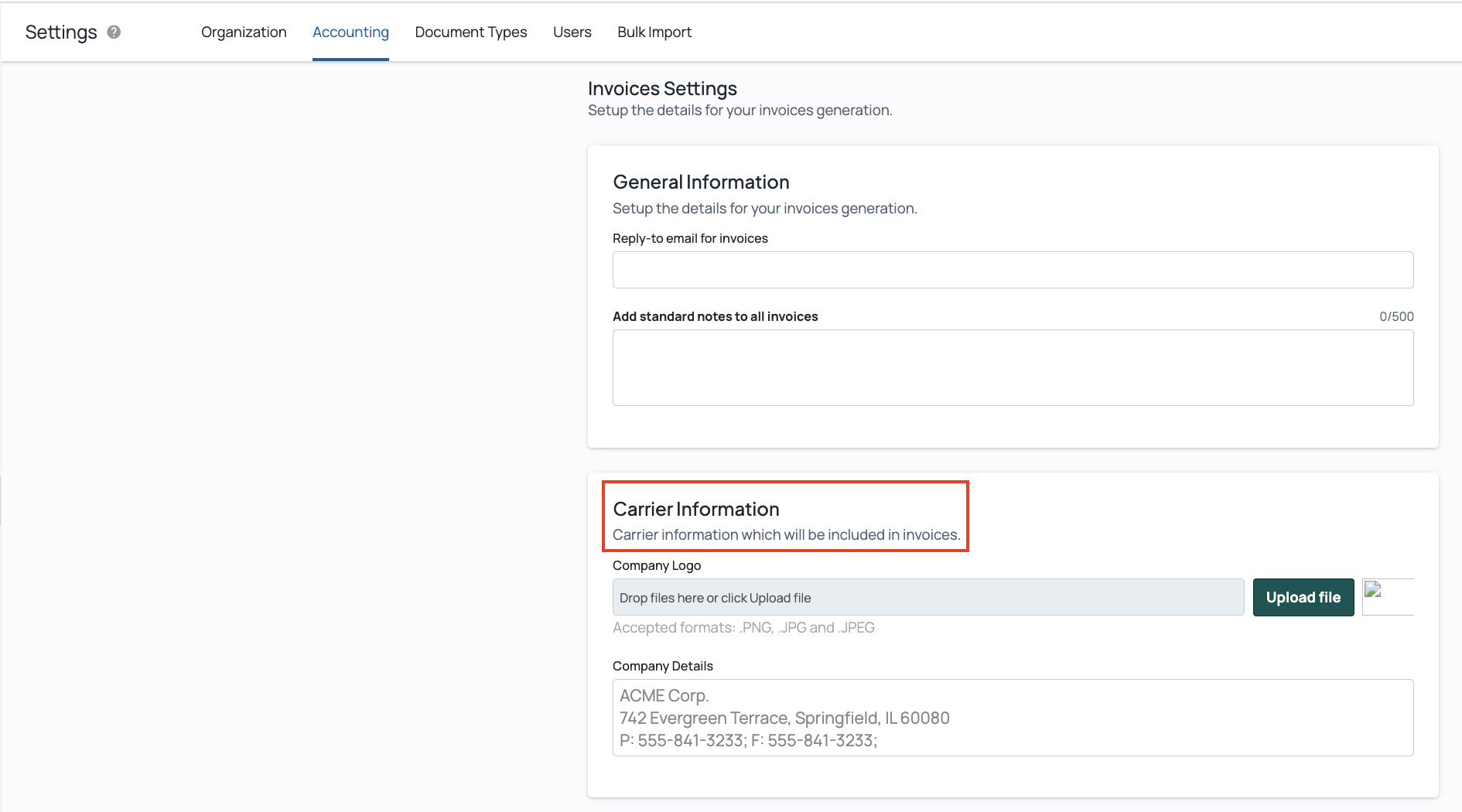# Invoice Settings

• Updated

This article will cover where to set remittance/pay to information on customer invoices.

### To set the invoice details:

1. On your left dashboard, go to Settings > General Settings >  Invoices2. Under Company Logo, upload the company logo image (accepted formats are .PNG, .JPEG. and .PDF).

3. Under Company Details, enter the remittance address and contact information (phone and/or fax).

5. Click Save Invoice Settings.

#### Related Articles:

0 out of 0 found this helpful

<% if (previousArticle || nextArticle) { %>
<% if (previousArticle) { %>
<% if (previousTitle) { %>

#### <%= previousTitle %>

<% } %> <%= previousArticle.title %>
<% } %> <% if (nextArticle) { %>
<% if (nextTitle) { %>

#### <%= nextTitle %>

<% } %> <%= nextArticle.title %>
<% } %>
<% } %>
<% if (allItems.length > 1) { %>

<% } %>
<% var getColumnClasses = function(numberColumns) { var classNames = 'col-12'; if (numberColumns >= 2) classNames += ' md:col-6'; if (numberColumns >= 3) classNames += ' lg:col-4'; if (numberColumns >= 4) classNames += ' xl:col-3'; return classNames; } %>

##<%= heading %>

<% } %>
<% blocks.forEach(function(block, index) { %>
• <% if (imageHeight) { %><% } %> <% if (block.name) { %>

## <%= block.name %>

<% } %> <% if (block.description) { %>

<%= block.description %>

<% } %>
• <% }) %>

##Featured articles

<% if (articles.length) { %>
<% articles.forEach(function(article) { %>
<%= Util.getExcerpt(article.body, 0) %>
<% }); %>
<% } else { %>

empty

<% } %>
<% var getColumnClasses = function(numberColumns) { var classNames = 'col-12 mb-4'; if (numberColumns >= 2) classNames += ' md:col-6'; if (numberColumns >= 3) classNames += ' lg:col-4'; if (numberColumns >= 4) classNames += ' xl:col-3'; return classNames; } %>

##<%= heading %>

<% } %>
<% blocks.forEach(function(block, index) { %>
• <% if (imageHeight) { %><% } %>
<% if (block.name) { %>

### <% if (block.html_url) { %> <%= block.name %> <% } else { %> <%= block.name %> <% } %>

<% } %> <% if (block.description) { %>

<%= block.description %>

<% } %>
• <% }) %>

Didn't find what you were looking for?

<% if (Theme.callToActionButtons && Theme.callToActionButtons.community.length) { %> <% Theme.callToActionButtons.community.forEach(function(button) { %> <% }) %> <% } %>
<% categories.forEach(function(category, index) { %>
• <% }); %>
<% var maxSections = 5 %>
<% if (sections.length) { %>
<% sections.forEach(function(section) { %>
• <%= partial('partial-article-list-sections', { id: 'section-' + section.id, parentId: '#' + id, sections: section.sections, activeCategoryId: activeCategoryId, activeSectionId: activeSectionId, activeArticleId: activeArticleId, partial: partial }) %> <% if (section.articles.length) { %> <% } %>
• <% }); %>
<% } %>
<% if (sections.length) { %>
<% sections.slice(0, maxSections).forEach(function(section) { %>
• <%= section.name %> <%= partial('partial-section-list-sections', { parent: section, sections: section.sections, maxSections: maxSections, partial: partial }) %>
• <% }); %> <% if (sections.length > maxSections) { %>
• See more
• <% } %>
<% } %>